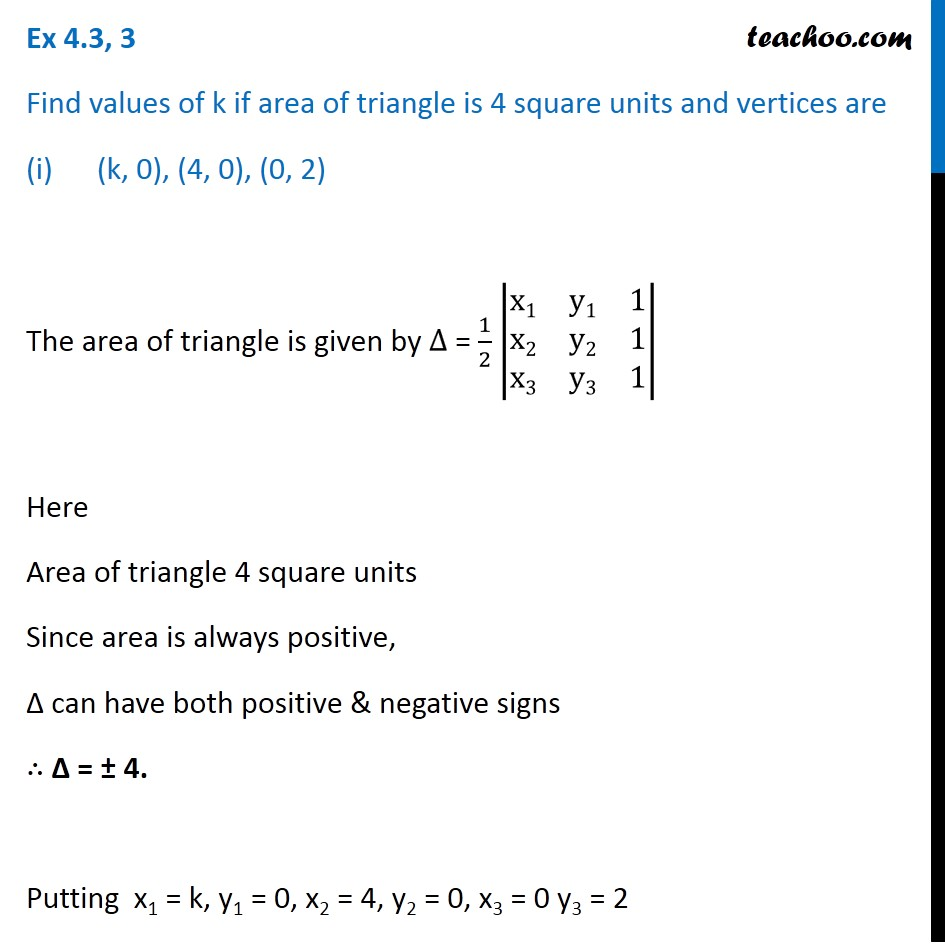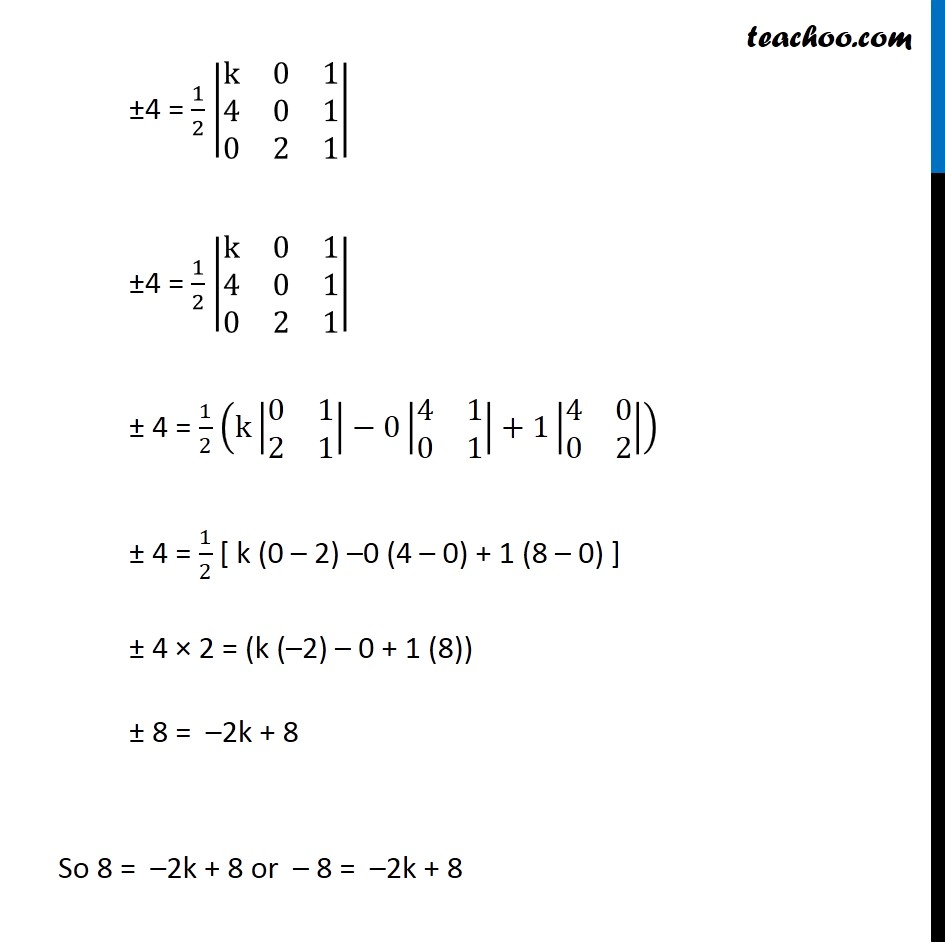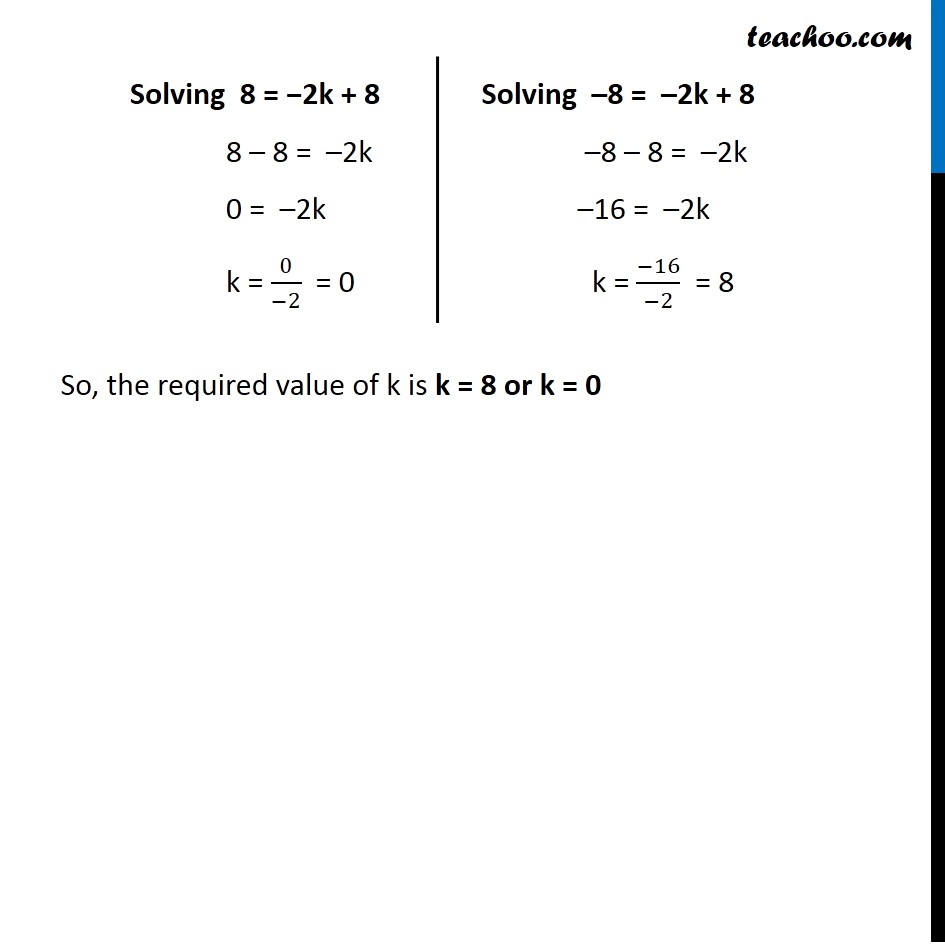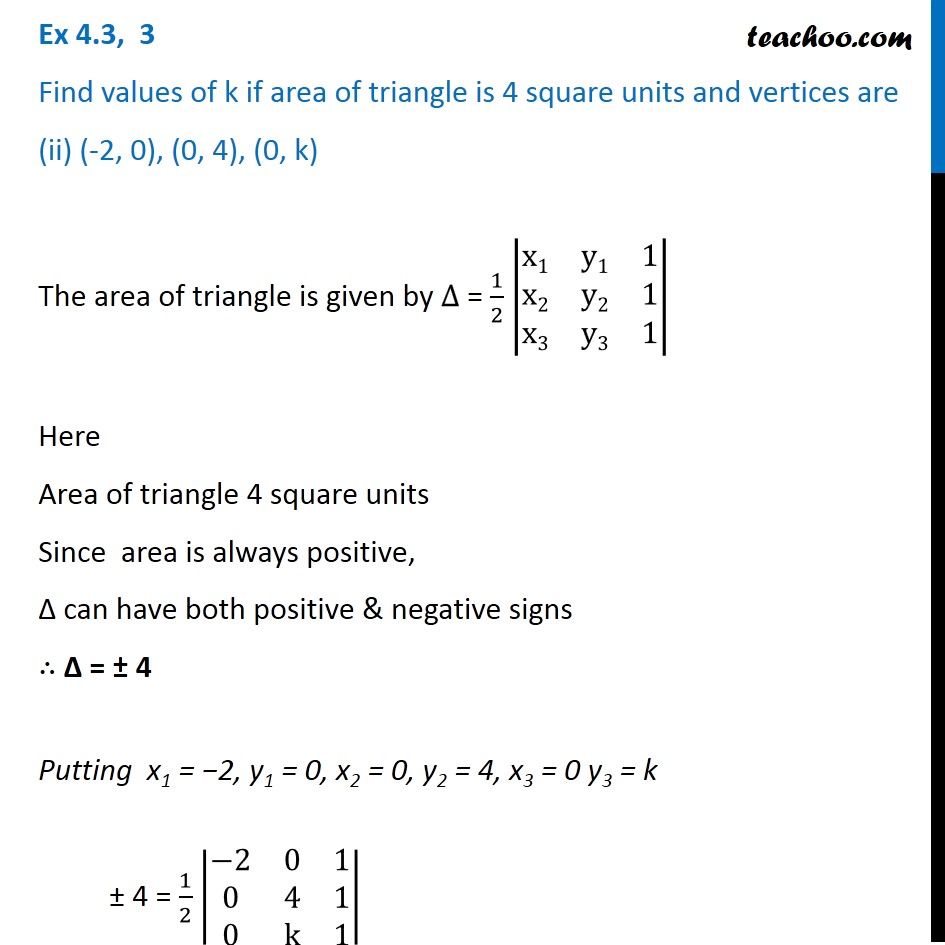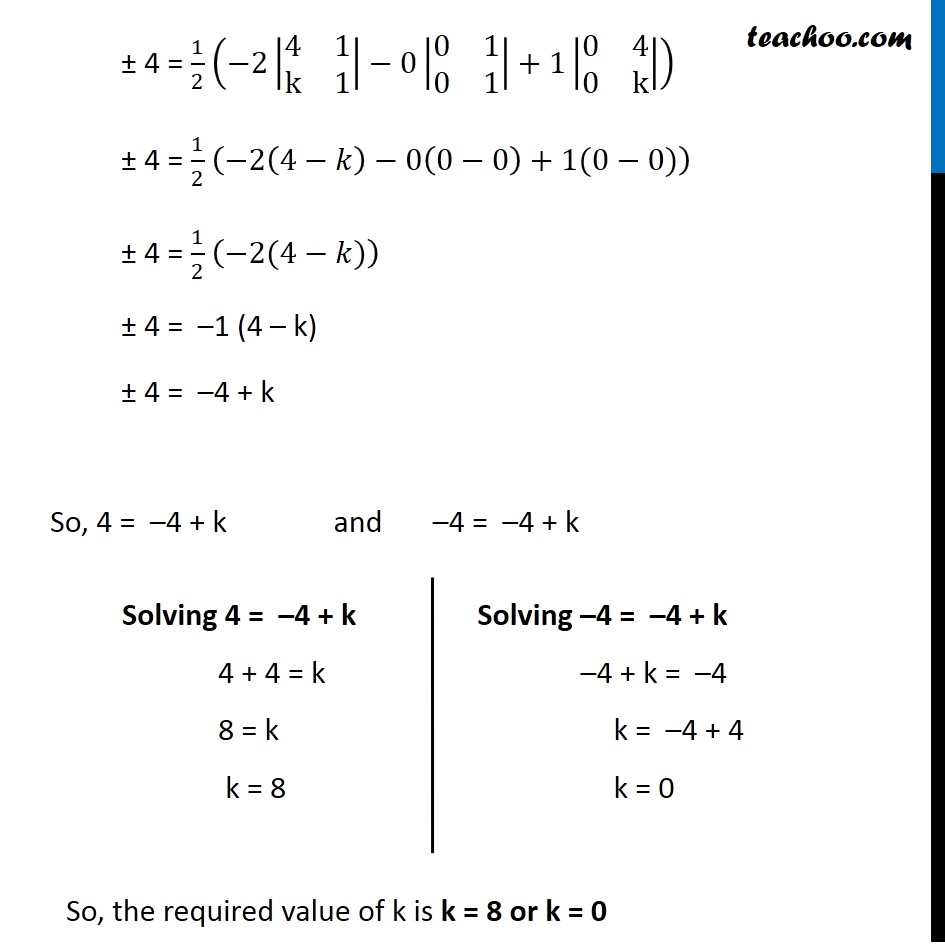Subscribe to our Youtube Channel - https://you.tube/teachoo

1. Chapter 4 Class 12 Determinants
2. Serial order wise
3. Ex 4.3

Transcript

Ex 4.3, 3 Find values of k if area of triangle is 4 square units and vertices are (k, 0), (4, 0), (0, 2) The area of triangle is given by ∆ = 1/2 |■8(x1&y1&1@x2&y2&1@x3&y3&1)| Here Area of triangle 4 square units Since area is always positive, ∆ can have both positive & negative signs ∴ Δ = ± 4. Putting x1 = k, y1 = 0, x2 = 4, y2 = 0, x3 = 0 y3 = 2 ±4 = 1/2 |■8(k&0&1@4&0&1@0&2&1)| ±4 = 1/2 |■8(k&0&1@4&0&1@0&2&1)| ± 4 = 1/2 (k|■8(0&1@2&1)|−0|■8(4&1@0&1)|+1|■8(4&0@0&2)|) ± 4 = 1/2 [ k (0 – 2) –0 (4 – 0) + 1 (8 – 0) ] ± 4 × 2 = (k (–2) – 0 + 1 (8)) ± 8 = –2k + 8 So 8 = –2k + 8 or – 8 = –2k + 8 Solving 8 = −2k + 8 8 – 8 = –2k 0 = –2k k = 0/(−2) = 0 +Solving –8 = –2k + 8 –8 – 8 = –2k –16 = –2k k = (−16)/(−2) = 8 So, the required value of k is k = 8 or k = 0 Ex 4.3, 3 Find values of k if area of triangle is 4 square units and vertices are (ii) (-2, 0), (0, 4), (0, k) The area of triangle is given by ∆ = 1/2 |■8(x1&y1&1@x2&y2&1@x3&y3&1)| Here Area of triangle 4 square units Since area is always positive, ∆ can have both positive & negative signs ∴ Δ = ± 4 Putting x1 = −2, y1 = 0, x2 = 0, y2 = 4, x3 = 0 y3 = k ± 4 = 1/2 |■8(−2&0&1@0&4&1@0&k&1)| ± 4 = 1/2 (−2|■8(4&1@k&1)|−0|■8(0&1@0&1)|+1|■8(0&4@0&k)|) ± 4 = 1/2 (−2(4−𝑘)−0(0−0)+1(0−0)) ± 4 = 1/2 (−2(4−𝑘)) ± 4 = –1 (4 – k) ± 4 = –4 + k So, 4 = –4 + k and –4 = –4 + k Solving 4 = –4 + k 4 + 4 = k 8 = k k = 8 Solving –4 = –4 + k –4 + k = –4 k = –4 + 4 k = 0 So, the required value of k is k = 8 or k = 0

Ex 4.3